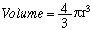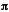home | O'Reilly's CD bookshelfs | FreeBSD | Linux | Cisco | Cisco Exam11.6. Creating Arrays of Scalar References

Problem

You want to create and manipulate an array of references to scalars. This arises when you pass variables by reference to a function to let the function change their values.

Solution

To create an array, either backslash each scalar in the list to store in the array:

@array_of_scalar_refs = ( \\$a, \\$b );

or simply backslash the entire list, taking advantage of the backslash operator's distributive property:

@array_of_scalar_refs = \( \$a, \$b );

To get or set the value of an element of the list, use \${ ... } :

\${ \$array_of_scalar_refs } = 12;         # \$b = 12

Discussion

In the following examples, @array is a simple array containing references to scalars (an array of references is not a reference to an array). To access the original data indirectly, braces are critical.

(\$a, \$b, \$c, \$d) = (1 .. 4);        # initialize
@array =  (\\$a, \\$b, \\$c, \\$d);     # refs to each scalar
@array = \( \$a,  \$b,  \$c,  \$d);     # same thing!
@array = map { \my \$anon } 0 .. 3;  # allocate 4 anon scalarresf

\${ \$array } += 9;                # \$c now 12

\${ \$array[ \$#array ] } *= 5;        # \$d now 20
\${ \$array[-1] }        *= 5;        # same; \$d now 100

\$tmp   = \$array[-1];                # using temporary
\$\$tmp *= 5;                         # \$d now 500

The two assignments to @array are equivalent  - the backslash operator is distributive across a list. So preceding a list (not an array) with a backslash is the same as applying a backslash to everything in that list. The ensuing code changes the values of the variables whose references were stored in the array.

Here's how to deal with such an array without explicit indexing.

use Math::Trig qw(pi);              # load the constant pi
foreach \$sref (@array) {            # prepare to change \$a,\$b,\$c,\$d
(\$\$sref **= 3) *= (4/3 * pi);   # replace with spherical volumes
}

This code uses the formula for deriving the volume of a sphere:The \$sref loop index variable is each reference in @array , and \$\$sref is the number itself, that is, the original variables \$a , \$b , \$c , and \$d . Changing \$\$sref in the loop changes those variables as well. First we replace \$\$sref with its cube, then multiply the resulting value by 4/3. This takes advantage of the fact that assignment in Perl returns an lvalue, letting you chain assignment operators together as we've done using the **= and *= assignment operators.

Actually, anonymous scalars are pretty useless, given that a scalar value fits in the same space as a scalar reference. That's why there's no explicit composer. Scalar references exist only to allow aliasing  - which can be done in other ways.11.5. Taking References to Scalars11.7. Using Closures Instead of Objects